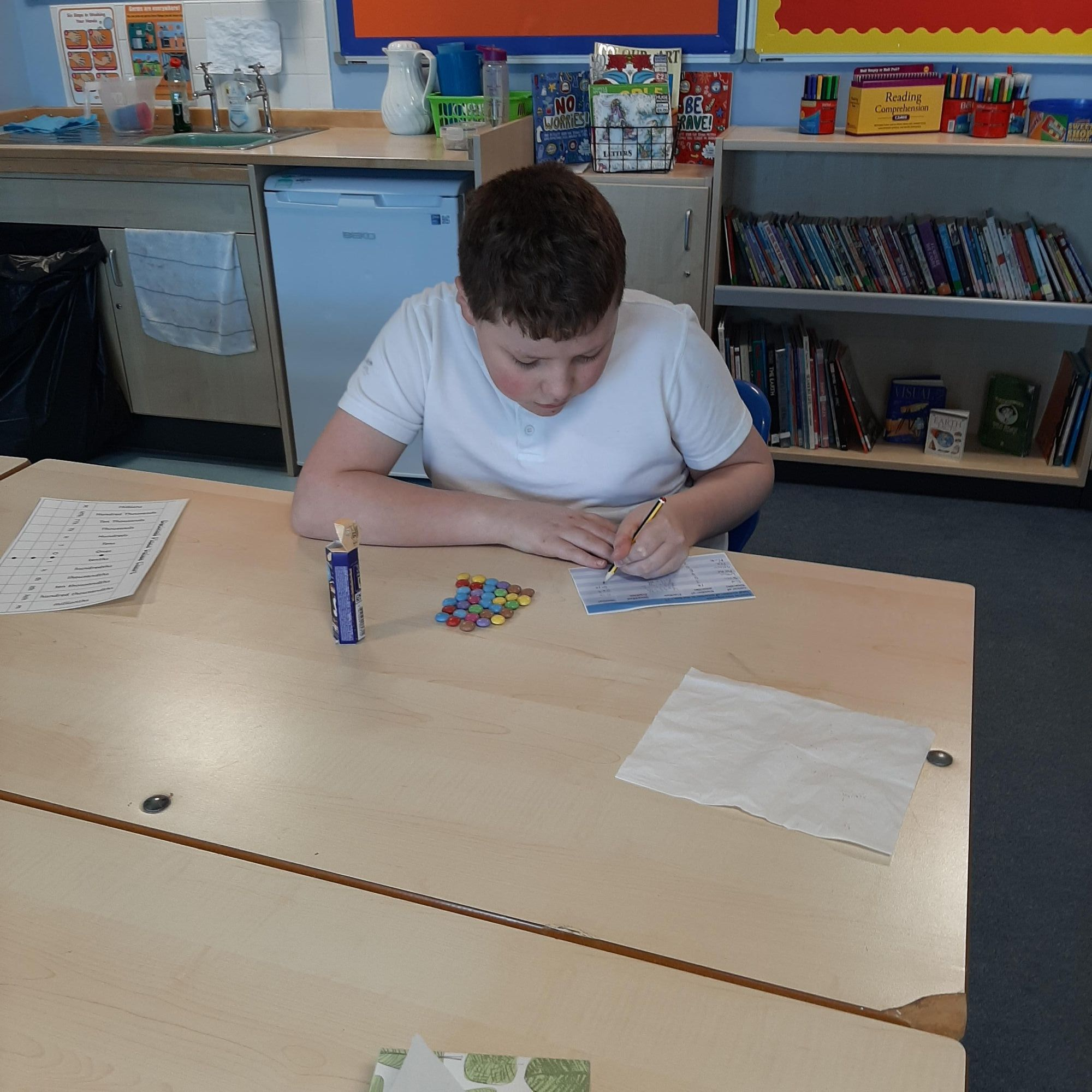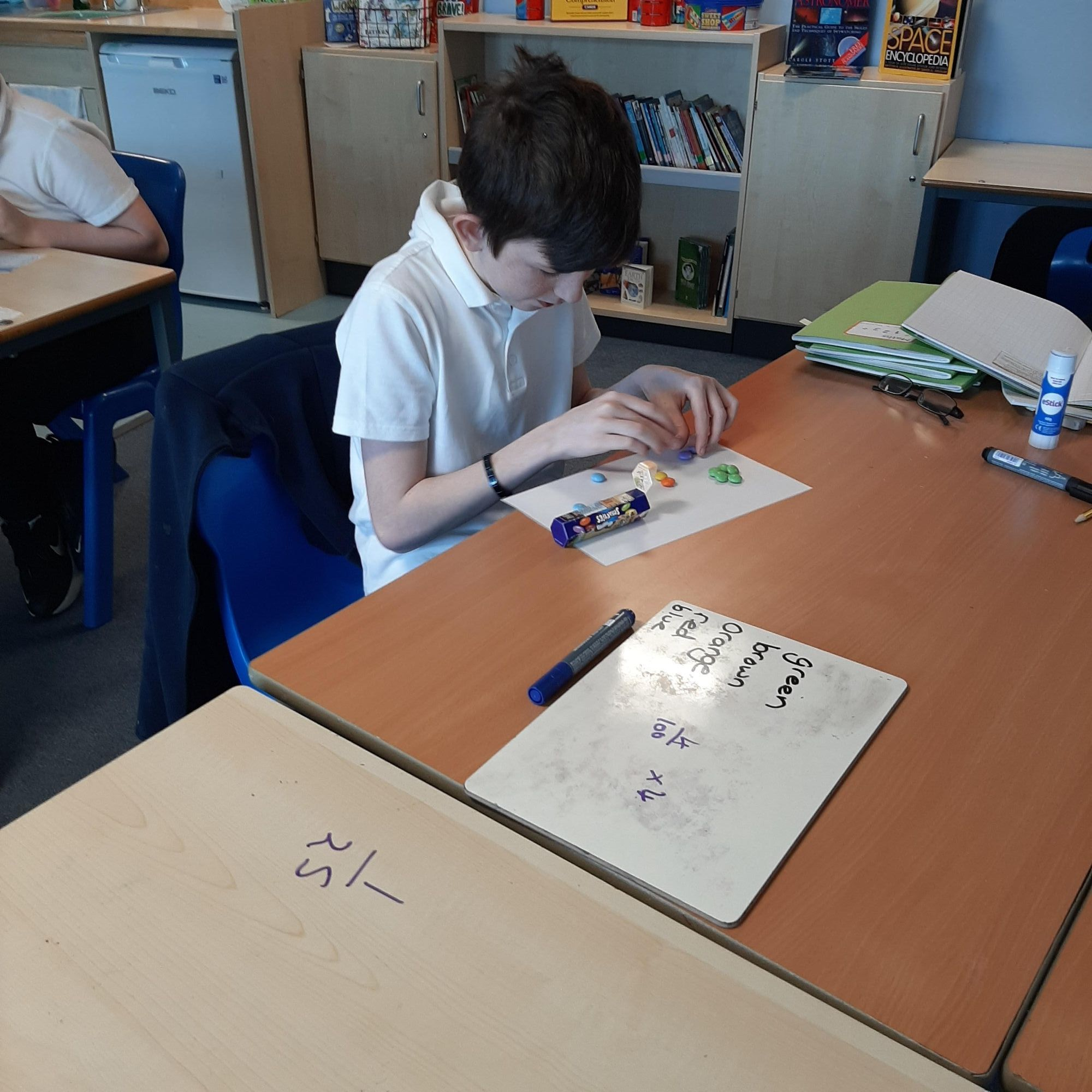# Fractions and DecimalsAlnmouth class have been studying Fractions and decimals in maths. We decided it would be much more fun if we completed one of our lessons practically, after all learning is much more relevant if we can complete hands on tasks.

We were each given a tube of smarties and had to count how many in total were in each box. We then needed to count how many of each colour were in each tube. We then needed to write these as fractions and some of us even managed to convert the fractions into decimals.

It was great fun and even better when we got to eat the smarties at the end.